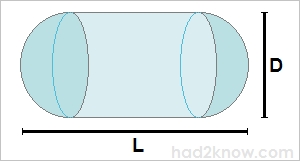# How to Calculate the Volume and Surface Area of a Capsule: Calculator and Formula

Capsule Volume & Surface Area
Diameter
Total Length
Volume =
Surface Area =A capsule is a solid geometric shape formed by placing two hemispheres at the ends of a cylinder. If you know the total length of the capsule from end to end and the diameter of the circular cross-section, you can use two simple formulas to compute its volume and surface area.

The equations are given below with an example, and you can also use the capsule calculator on the left.

### Finding the Volume

If the total length of the capsule is L and the diameter of the cylinder and hemispherical ends is D, then the length of the cylinder in the middle is L - D. Let C = L - D. The volume of the cylindrical portion of the capsule is πCD²/4. The total volume of the two hemispherical ends is the volume of a whole sphere with a diameter of D, thus πD³/6. Therefore, the total volume of the capsule is

πCD²/4 + πD³/6

= π(L-D)D²/4 + πD³/6

= πLD²/4 - πD³/12

= πD²(3L-D)/12.

### Finding the Surface Area

The surface area of the cylinder in the middle is πCD. The combined surface area of the two hemispheres is πD². Thus, the entire surface area of the capsule is

πCD + π

= π(L-D)D + π

= πLD.

### Example

A tank shaped like a capsule has a total length of 3.2 meters and a diameter of 1.4 meters. Since L = 3.2 and D = 1.4, the volume is

π1.4²(3(3.2)-1.4)/12 = 4.2076 m³ = 4207.6 L

The surface area is

π(1.4)(3.2) = 14.0743 m²